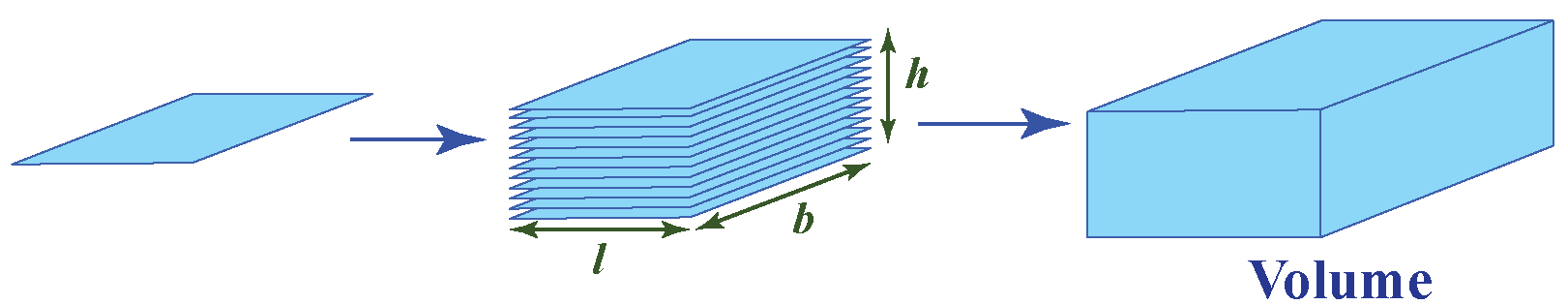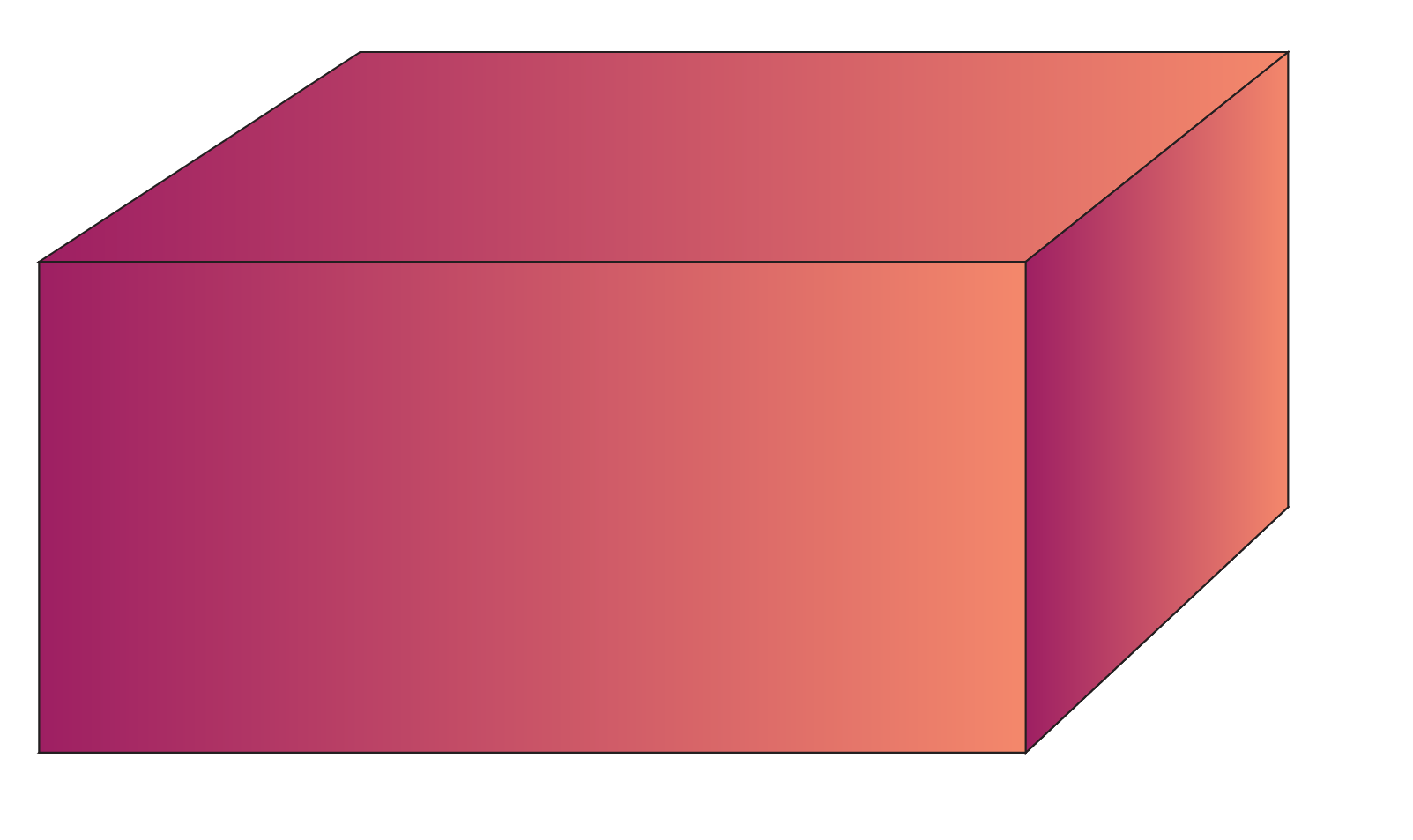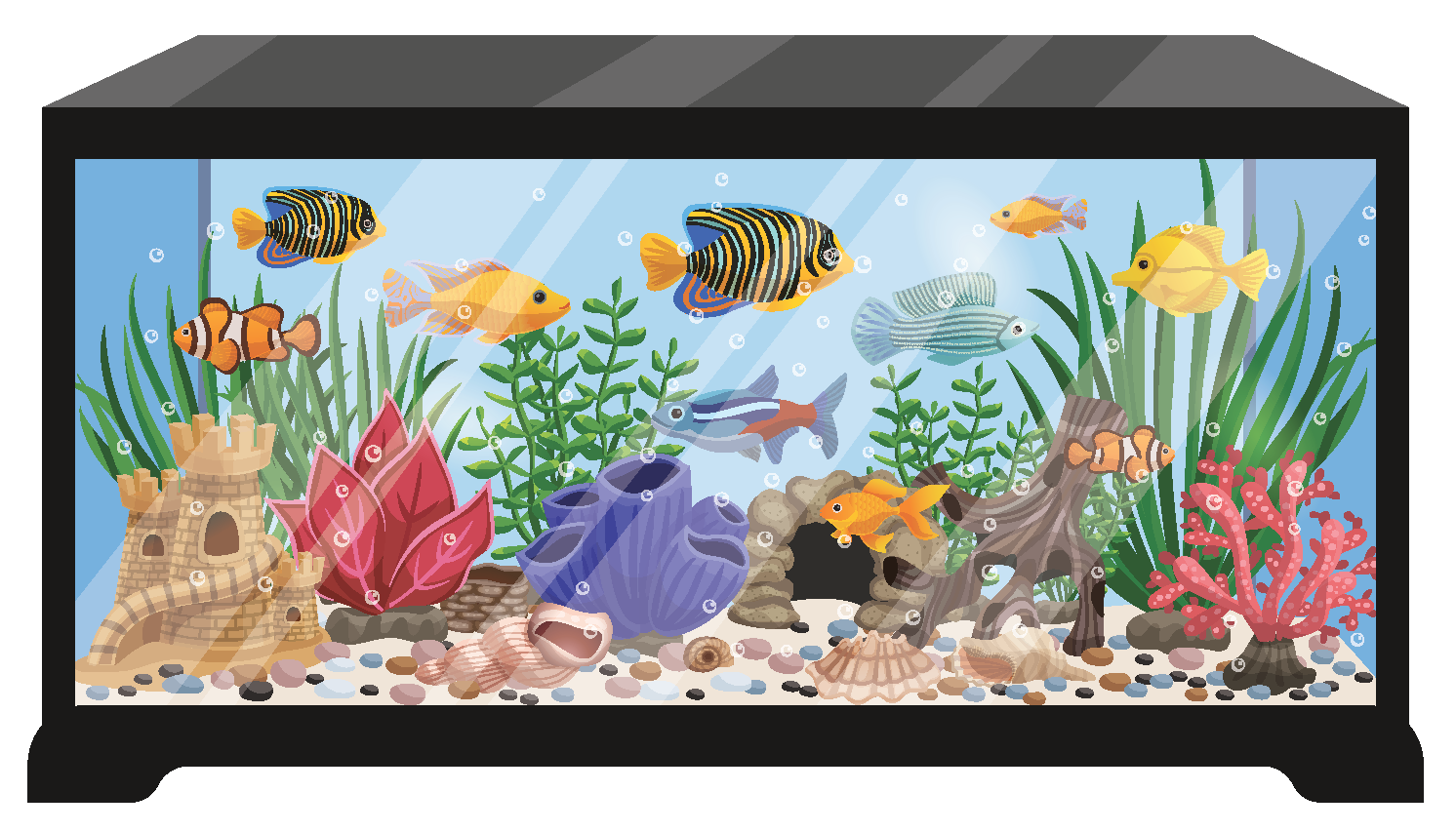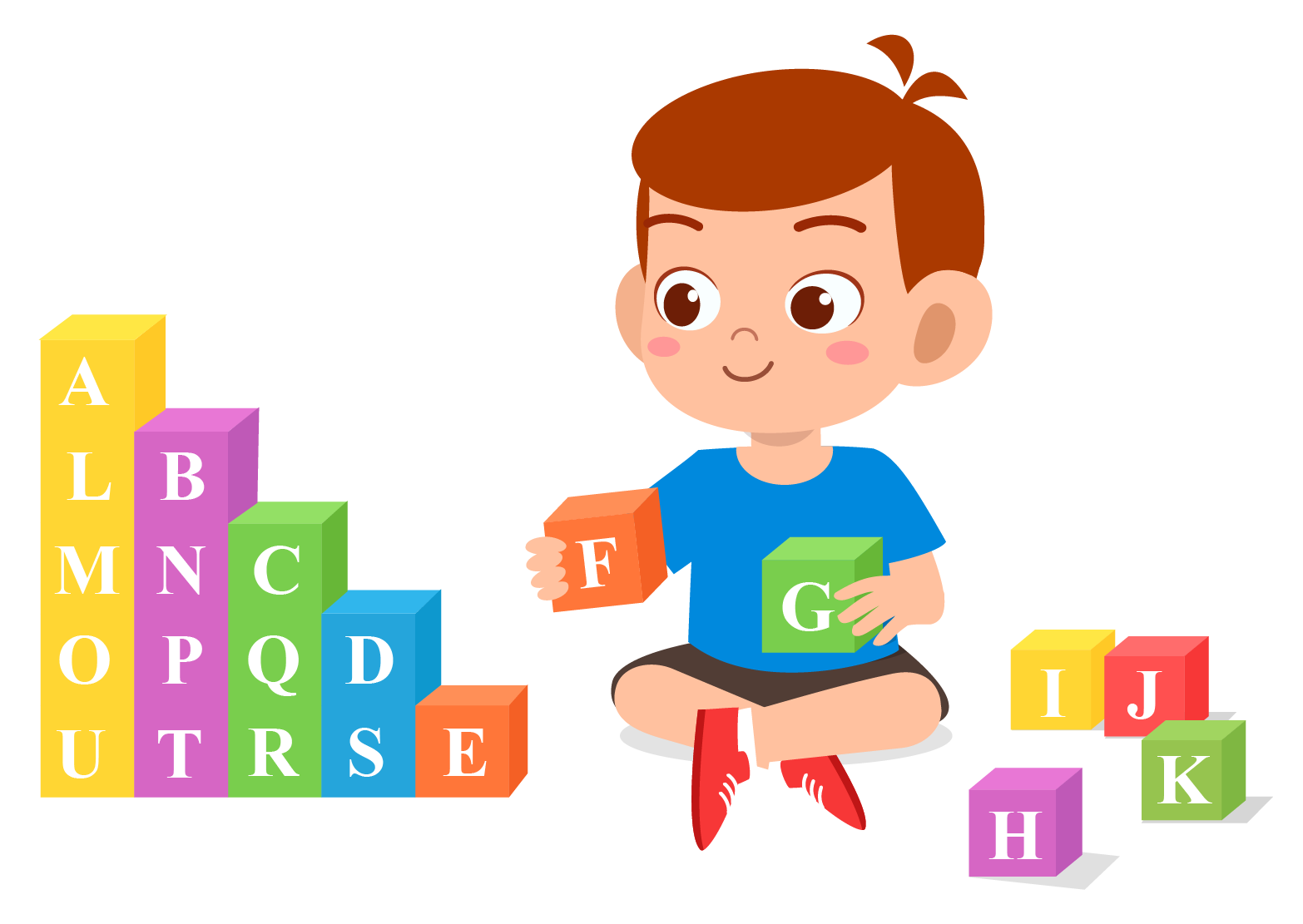# Volume of a cuboid

Volume of a cuboid
Go back to  'Volume'

Hey kids! Take a minute to look around your room.

Do you see anything in your room which is in the shape of a rectangular box?It could be anything like a book, gift box, or a brick.

Do you know what this shape is called?

This shape is cuboid and it is extremely a common shape to see around us.

In this short lesson, let us learn about how to find the volume of a cuboid. Once you understand this chapter and the monomial rules, you will learn how to solve problems on the volume of cuboid without using a volume of a cuboid calculator.

## Lesson Plan

 1 What Is Volume? 3 Important Notes on Volume of Cuboid 4 Solved Examples on Volume of Cuboid 2 Thinking Out of the Box! 5 Interactive Questions on Volume of Cuboid

## What Is Volume?

Katie and her children are making clay models of some solid shapes.One thing common in all these solids is that they have some length, breadth, and height.

They all occupy space and have 3 dimensions.

The amount of clay that these shapes occupy is called its volume.

So, the volume is the space occupied by any solid shape.

## What Is the Volume of a Cuboid?

What will happen if you stack a bundle of many sheets of paper?

How does it look?

It makes up a cuboid?### Volume of a Cuboid Formula

Let the area of a rectangular sheet of paper be $$A$$, the height up to which they are stacked be $$h$$ and the volume of the cuboid be $$V$$.

Then, the volume of the cuboid is given by multiplying the base area and height.

$\text{Volume of Cuboid}=\text{Base Area} \times \text{Height}$

 $$\text{Volume of Cuboid}=lbh$$

## How to Calculate Volume of a Cuboid?

Let's calculate the volume of a cuboid using its formulaLet $$l=7\;\text{in}$$, $$b=5\;\text{in}$$ and $$h=2\;\text{in}$$

\begin{align}\text{Volume of Cuboid}&=lbh\\&=(7\times5\times 2)\;\text{in}^{3}\\&=70\;\text{in}^{3}\end{align}

### Volume Of a Cuboid Calculator

Experiment with the calculator below to find the volume of a cuboid.

Change the sliders for length, breadth, and height to change the dimensions.

We hope this volume of a cuboid calculator served you really well!

## Solved Examples

 Example 1

Mariam got this beautiful fish aquarium.The dimensions of the fish aquarium are $$30\text{ in.}$$, $$20\text{ in.}$$ and $$15 \text{ in.}$$.

Can you determine the volume of the fish aquarium?

Solution

The fish aquarium is of cuboidal shape.

Length of the aquarium =  $$30\text{ in.}$$

Width of the aquarium = $$20\text{ in.}$$

Height of the aquarium = $$15\text{ in.}$$

Therefore, the volume of the aquarium is:

\begin{aligned}\text{Volume}&=\text{Length}\times\text{Width}\times\text{Height}\\&=30\;\text{in}\times20\;\text{in}\times15\;\text{in}\\&=9000\text{ in}^{3}\end{aligned}

 So, the volume of the aquarium is $$9000\text{ in}^{3}$$.
 Example 2

Sally wants to buy a soft drink.She bought a cuboidal can with length $$20\;\text{in}$$, breadth $$15\;\text{in}$$ and height $$10\;\text{in}$$.

Sally wants to find the volume of this can.

Can you help her?

Solution

Let's calculate the volume of the can.

Volume of Cuboid:

\begin{align}\text{Volume}&=\text{Length} \times \text{Breadth} \times \text{Height}\\&=20\;\text{in} \times 15\;\text{in}\times 10\;\text{in}\\&=3000\;\text{in}^{3}\end{align}

 $$\therefore$$ The volume of the can is $$3000\;\text{in}^{3}$$.Think Tank
 Joe loves playing with building blocks. He has built a structure as shown below. The edge of each cube is 3 inches.1. Can you calculate the height of the green cuboid? 2. Can you find the sum of volumes of yellow and purple cuboids?

## Interactive Questions

Here are a few activities for you to practice. Select/type your answer and click the "Check Answer" button to see the result.

## Let's Summarize

This mini-lesson targeted the fascinating concept of volume of cuboid. The math journey around the volume of cuboid starts with what a student already knows, and goes on to creatively crafting a fresh concept in the young minds. Done in a way that is not only relatable and easy to grasp but will also stay with them forever. Here lies the magic with Cuemath.

At Cuemath, our team of math experts is dedicated to making learning fun for our favorite readers, the students!

Through an interactive and engaging learning-teaching-learning approach, the teachers explore all angles of a topic.

Be it worksheets, online classes, doubt sessions, or any other form of relation, it’s the logical thinking and smart learning approach that we, at Cuemath, believe in.

## 1. How to find the volume of a cuboid?

The volume of a cuboid is calculated by multiplying its length, width, and height.

## 2. What is the formula for the volume of a cuboid?

The formula of volume of a cuboid is = $$\text{Length}\times \text{Width}\times \text{Height}$$.

## 3. If the units of dimensions of a cuboid are different, then how to find the volume?

If the units of dimensions of a cuboid are different, then first we need to change the units of dimensions of any two dimensions in the unit of the third dimension.

After that, multiply all three dimensions to calculate the volume of the cuboid.

## 4. Does the order of height, width, and length matter while calculating the volume of a cuboid?

No, the order of height, width, and length does not matter because we need to multiply all three quantities to determine the volume of a cuboid.

Mensuration and Solids
Mensuration and Solids
grade 10 | Questions Set 2
Mensuration and Solids